# Compare 2 Columns Through VBA in Microsoft Excel

In case you are wondering how to compare 2 columns of data having rows in millions & extract the unique values among both the columns then you should read this article. We will use VBA code to compare two columns of data & show the difference in next two columns.

Question: There are multiple values which exist in columns A & B. I want a macro to check more than 40 k rows of data in both of the columns & then extract the list of unique entries from each of the column into the next column i.e. columns C & D respectively. In this example, I am taking only 40 rows of sample data.

You can find original question here

Following is the snapshot of data: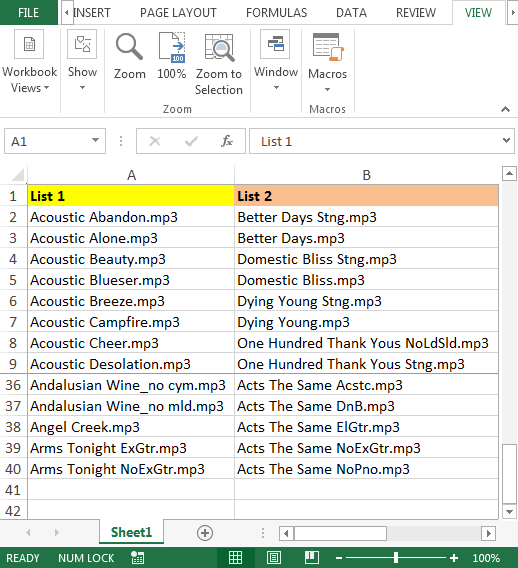To compare the list of two columns, we need to follow the below steps to launch VB editor:

• Click on Developer tab
• From Code group select Visual Basic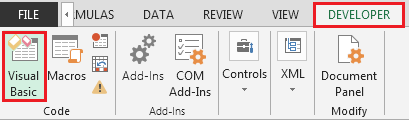• Copy the below code in the standard module

```Sub PullUniques()
Dim rngCell As Range
For Each rngCell In Range("A2:A40")
If WorksheetFunction.CountIf(Range("B2:B40"), rngCell) = 0 Then
Range("C" & Rows.Count).End(xlUp).Offset(1) = rngCell
End If
Next
For Each rngCell In Range("B2:B40")
If WorksheetFunction.CountIf(Range("A2:A40"), rngCell) = 0 Then
Range("D" & Rows.Count).End(xlUp).Offset(1) = rngCell
End If
Next
End Sub
```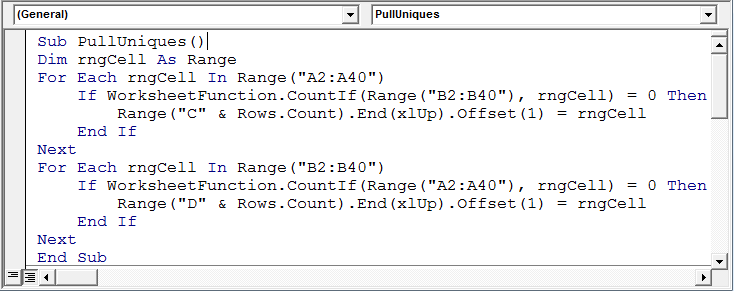• The above macro is all set to run; either we can press F5 key –if you are currently in Visual Basic Editor Screen –or we can use shortcut keys “ALT + F8”, select your macro & click on run button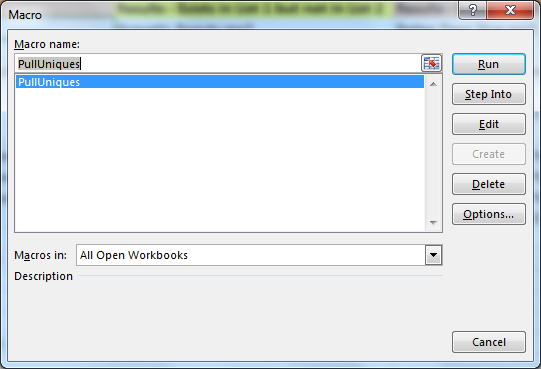• The unique list from columns A & B will be generated automatically in columns C & D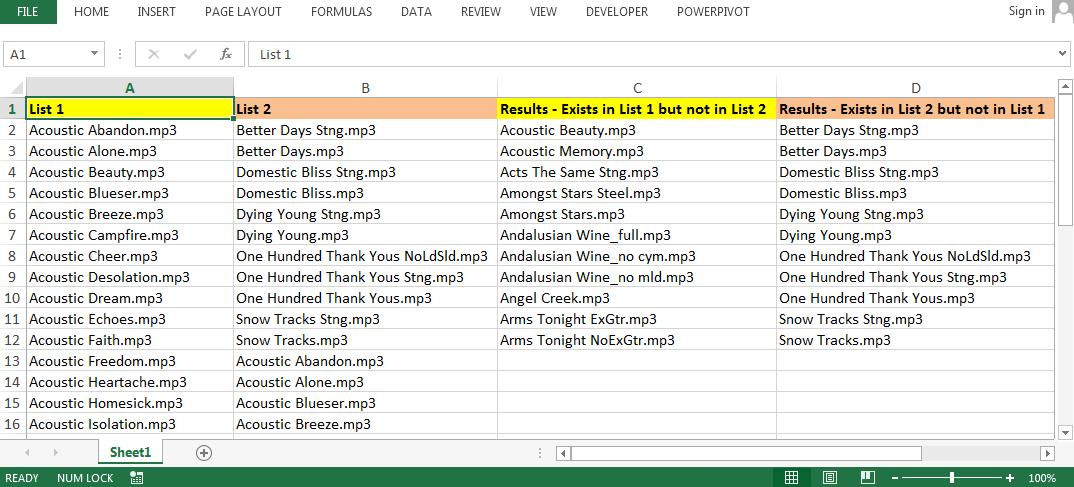Code Explanation:

In the above code, we will use IF function along with For loop to get the result.

• We declare Dim rngCell As Range; the purpose of declaring rngCell As Range is we want to assign the sample range “A2:A40” with a specific name i.e. “rngCell” in our example
• For Each rngCell In Range("A2:A40"); we will check for each cell in “rngCell” with a condition
• If WorksheetFunction.CountIf(Range("B2:B40"), rngCell) = 0 Then; this line of code is using COUNTIF VBA function to check criteria range B2:B40 with criteria rngCell is equal to zero, then in column C, the value stored in rngCell will be saved
• Similarly, we will run For Each Loop for column B & extract the unique values in column D

Conclusion: We can get the unique list of text or values from each of the column; the header name of the column C (Results - Exists in List 1 but not in List 2) & column D (Results - Exists in List 2 but not in List 1). In case we have similar requirement but the number of columns are more than two then we need to tweak the code in order to get the result.

We would love to hear from you, do let us know how we can improve, complement or innovate our work and make it better for you. Write us at info@exceltip.com

1.VIPUL D. JHAVERI

THANKS FOR HIGHLY USEFUL TIP
FOR VALUE COMING TWICE AND MORE , WE HAVE TO ADD CHECK-COUNT AND GENERATE UNIQUE KEY VALUE
WE CAN USE COUNTIF , E.G. 18000 IS REPEATED AT ROW 12 AND ROW 24 IN COLA, =COUNTIF(\$A\$1:A12,A12) WILL RETURN 1 , =COUNTIF(\$A\$1:A24,A24)
WILL RETURN 2 AND WITH "&" USE, WE CAN ADD BASIC VALUE AND COUNT VALUE TO GET UNIQ VALUE FOR BOTH COMPARATIVE COLUMNS
SO THIS HELP TO KNOCK OFF RELEVANT OCCURRENCES ONLY

2.Hi My excel columns has values like the following:

Column A1 : 1234,2345,6789,4578 and Column B1 : 2345,7896,3452,1234 ,9887.Can I possibly compare and find out how many are missing in B1 and what are they ?
Like in this case 2/4 and they missing in B1 are 6789,4578 .

3.Hi, thanks for your help. I want to compare two columns with values and get the values that don't match but sometimes I have a column with the same value twice and the result shows that this value exists in column 2 while it exists only once in column 2. Could you please help?

4.I am use your -Compare-2-Columns-Show-differences-Through-VBA its good but some problem in the file use for me. Problem (1) Same Value may time in (A) First column but same value only one time in Column (B) The result Show Actual in Difference in result column not match.
My Result is Show column A Same Value compare in B only Once time do note compare many time Than Show pending value in Result Column. I Use this File in Accounting maters in many value.

5.I had a "Compare Columns.xla" add in Macro that worked in Office 2010 - but it does not work in Excel 2016 (Crashes Excel). Do you have instructions for creating a compare columns macro in Excel 2016? It would be much appreciated!

6.Thank you for your lesson. If it is possible, can you PLEASE, explained how to compare 5 tables in different sheets, I need to compare Row by Row, I have a 5 spreadsheet with dynamic arrays each one like B2:F36. Thank you.

7.•Replace the range with this:
Range("A1", Range("A1").End(xlDown))
•